# 10.2.3 Circles I, PT3 Focus Practice

            
Question 11:
  
The Town Council plans to build an equilateral triangle platform in the middle of a roundabout. The diameter of circle RST is 24 m and the perpendicular distance from R to the line ST is 18 m. as shown in Diagram below.Find the perimeter of the platform.
  
  Solution:Given diameter = 24 m
  hence radius = 12 m
  O is the centre of the circle.
  Using Pythagoras’ theorem:
            
  
 
      
Question 12:
 
Amy will place a ball on top of a pillar in Diagram below. Table below shows the diameters of three balls X, and Z.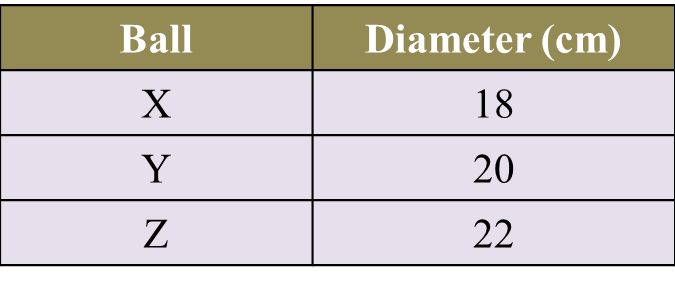Which ball X, or Z, can fit perfectly on the top of the pillar? Show the calculation to support Amy’s choice.
 
 Solution:Question 13:
 
Diagram below shows a rim of a bicycle wheel with a diameter of 26 cm. Kenny intends to build a holder for the rim.Which of the rim holder, X, or Z, can fit the bicycle rim perfectly? Show the calculation to support your answer.
 
 Solution: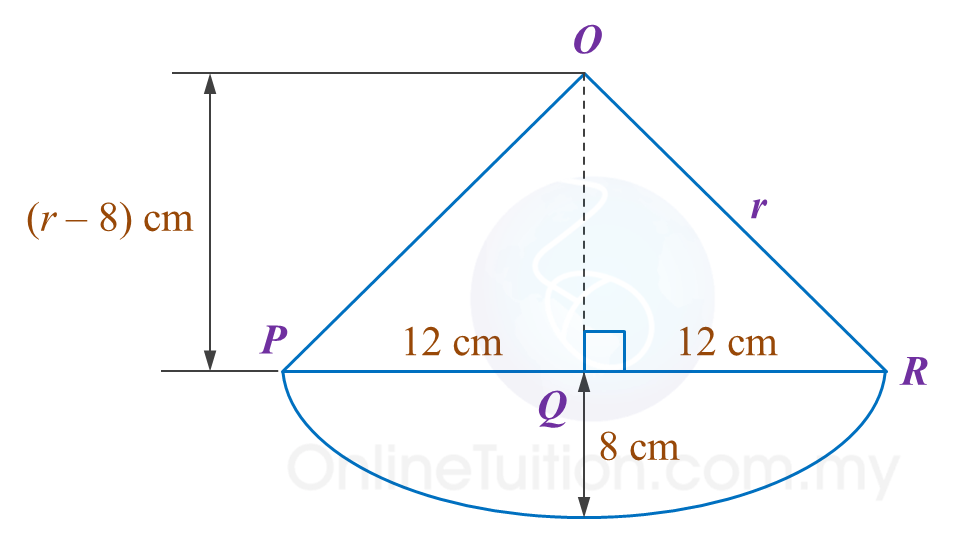# 10.2.2 Circles I, PT3 Focus Practice

                       
Question 6:
       In the diagram below, CD is an arc of a circle with centre O.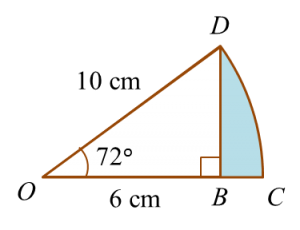Determine the area of the shaded region.
                                                    
 
 Solution:

                                             
      
    
      
                   
 Question 7:
 In diagram below, ABC is a semicircle with centre O.Calculate the area, in cm2 , of the shaded region.
                                     
 
   Solution:
                                         
 
 
                   
 Question 8:
 In diagram below, ABC is an arc of a circle centre O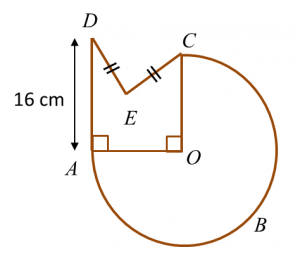The radius of the circle is 14 cm and AD = 2 DE.
   Calculate the perimeter, in cm, of the whole diagram.
                                       
 
    Solution:
                                       
 
 
 [adinserter block="3"]                
Question 9:
 
In diagram below, KLMN is a square and KLON is a quadrant of a circle with centre K.Calculate the area, in cm2, of the coloured region.
                     
 
 Solution:
               
 
 
 
 [adinserter block="3"]                
Question 10:
 
Diagram below shows two quadrants, AOC and EOD with centre O.Sector AOB and sector BOC have the same area.
 Calculate the area, in cm2, of the coloured region.
                     
 
 Solution:
               
 
 
 

# 10.2.1 Circles I, PT3 Focus Practice

10.2.1 Circles I, PT3 Focus Practice

Question 1:
Diagram below shows a circle with centre O.

The radius of the circle is 35 cm.
Calculate the length, in cm, of the major arc AB.

Solution:
Angle of the major arc AB = 360o – 144o= 216o
$\begin{array}{l}\text{Length of major arc}AB\\ =\frac{{216}^{o}}{{360}^{o}}×2\pi r\\ =\frac{{216}^{o}}{{360}^{o}}×2×\frac{22}{7}×35\\ =132\text{cm}\end{array}$

Question 2:
In diagram below, O is the centre of the circle. SPQ and POQ are straight lines.

The length of PO is 8 cm and the length of POQ is 18 cm.
Calculate the length, in cm, of SPT.

Solution
:
Radius = 18 – 8 = 10 cm
PT2 = 102 – 82
= 100 – 64
= 36
PT = 6 cm
Length of SPT = 6 + 6
= 12 cm

Question 3:
Diagram below shows two circles. The bigger circle has a radius of 14 cm with its centre at O.
The smaller circle passes through O and touches the bigger circle.

Calculate the area of the shaded region.

Solution:

Question 4:
Diagram below shows two sectors. ABCD is a quadrant and BED is an arc of a circle with centre C.

Calculate the area of the shaded region, in cm2.

Solution
:

Question 5:
Diagram below shows a square KLMN. KPN is a semicircle with centre O.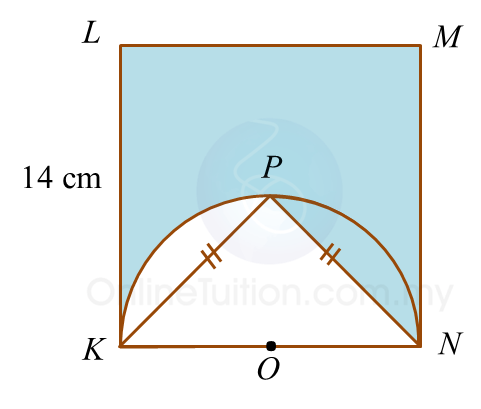Calculate the perimeter, in cm, of the shaded region.

Solution
:

= KL + LM + MN + NP + Arc length PK
= 14 + 14 +14 + 9.90 + 11
= 62.90 cm

# 10.1 Circles I

     
   
10.1 Circles I
   

   
10.1.1 Parts of a Circle
   
1. A circle is set of points in a plane equidistant from a fixed point.
   

   2.
Parts of a circle:
   
(a)    The centre, O, of a circle is a fixed point which is equidistant from all points on the circle.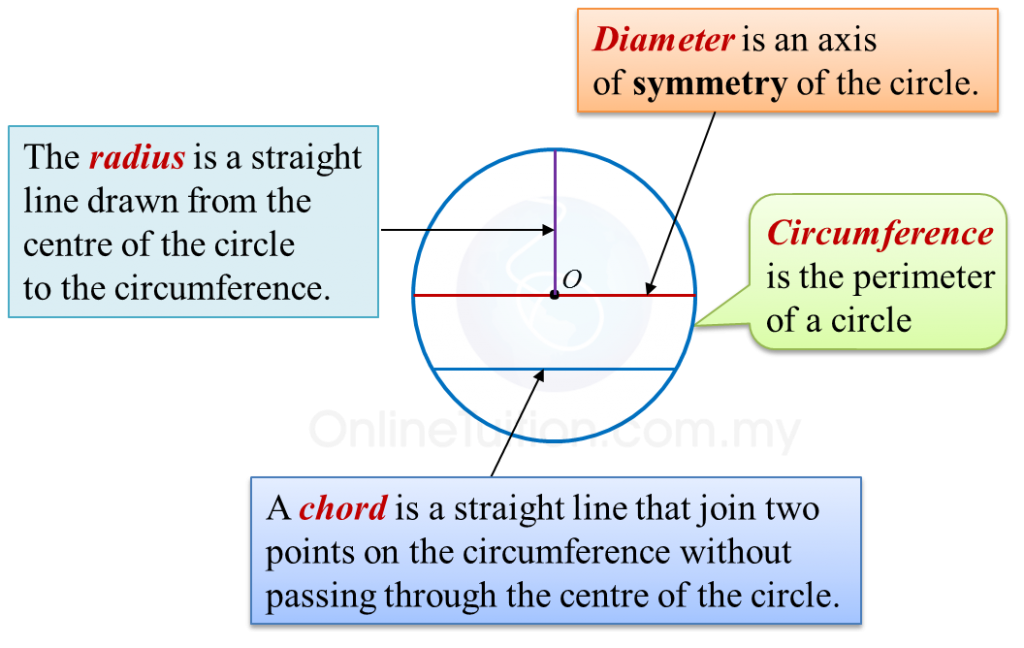(b)
A sector is the region enclosed by two radii and an arc.
   
   
   
       
 
     

   
   (c)
An arc is a part of the circumference of a circle.
       
   

   
   (d)
A segment is an area enclosed by an arc and a chord.
   

   
   
       
 
 
     
   
10.1.2 Circumference of a Circle
   
           
     
Example:
   
Calculate the circumference of a circle with a diameter of 14 cm.     $\left(\pi =\frac{22}{7}\right)$    
   
   

    Solution
:
      $\begin{array}{l}\text{Circumference}=\pi ×\text{Diameter}\\ \text{}=\frac{22}{7}×14\\ \text{}=44\text{cm}\end{array}$

   

   
 
     
   
10.1.3 Arc of a Circle
   
The length of an arc of a circle is proportional to the angle at the centre.
           
     
Example:
   
       

   
Calculate the length of the minor arc AB of the circle above.     $\left(\pi =\frac{22}{7}\right)$    
   

    Solution
:
   
    $\begin{array}{l}\frac{\text{Length of arc}}{\text{Circumference}}=\frac{\text{Angle at centre}}{{360}^{o}}\\ \\ \text{Length of arc}AB=\frac{{120}^{o}}{{360}^{o}}×2×\frac{22}{7}×7\\ \text{}=14\frac{2}{3}\text{cm}\end{array}$

   
   
 
 
     
   
10.1.4 Area of a Circle
   
   
   
   
         
   

   
Example:
   
Calculate the area of each of the following circles that has
   
(a) a radius of 7 cm,
   
(b)   a diameter of 10 cm.
   
    $\left(\pi =\frac{22}{7}\right)$    
   

    Solution
:
   
(a)
   
    $\begin{array}{l}\text{Area of a circle}=\pi {r}^{2}\\ \text{}=\frac{22}{\overline{)7}}×\overline{)7}×7\\ \text{}=154{\text{cm}}^{2}\end{array}$    
 
     
(b)
   
    $\begin{array}{l}\text{Diameter of circle}=10\text{cm}\\ \text{Radius of circle}=5\text{cm}\\ \text{Area of circle}=\pi {r}^{2}\\ \text{}=\frac{22}{7}×5×5\\ \text{}=78.57{\text{cm}}^{2}\end{array}$

   
 
 
     
   
10.1.5 Area of a Sector
   
The area of a sector of a circle is proportional to the angle at the centre.
   
         
   

    Example
:
       

   
   

   
   
   
   
   
        $\begin{array}{l}\text{Area of sector}ABC\\ =\frac{{72}^{o}}{{360}^{o}}×\frac{22}{7}×7×7\\ =30\frac{4}{5}{\text{cm}}^{2}\end{array}$